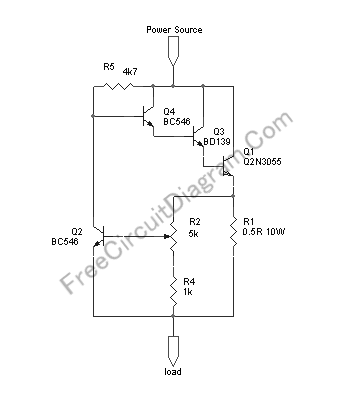# Variable (Adjustable) Current Limiter CircuitThis circuit provide automatic current limiting up to 8.4A. Unlike current limiter that uses only a resistor, this current limiting circuit doesn’t drop the voltage, or at least keep the voltage drop at minimum, until a certain current amount is exceeded. This current amount limit is adjustable from 1.4 A to 8.4A using a potentiometer. You can modify the component value to give different current limiting range. Here is the circuit’s schematic diagram:The resistor R1 is there to sense the current. At R2 potentiometer at minimum resistance (the center tap connected to R1), if the current drawn by the load reach 1.2A then the voltage across R1 reach 0.6V and Q2 begin conducting, thus shorting the base voltage of Q4 to ground. This shorting action reduce the base current and therefore reduce the output voltage sensed by the load, and prevent the current to flow further. If you need the current limiter to limit at lower threshold range, you can change the R1 to 1R and you’ll get about 0.7A to 4.2A adjustment range.

Because of the power dissipation capability of 2N3055 transistor, at the worst case that the load is shorted to ground (zero resistance), if you limit the current to 8.4 A then the circuit can handle maximum source voltage of 14V, while limiting the current at 4.2A can handle up to 27V source voltage. The maximum voltage can be handled by this circuit is 60 volt, but at that maximum voltage you can only safely set the current limit at 1.9A in the extreme condition, when the load is shorted to ground. Please make sure the Q1 transistor has sufficient heat sink. [Circuit Schematic Source: Designed by FreeCircuitDiagram.Com]

Update (some questions from our reader)
I have some questions regarding this circuit:

1. How is the input ground and the output ground connected in this circuit?  I’m guessing that the ground is connected from the load to he power source, but that would not be true if this is intended to be a two input/ two output device.
2. If my load is equivalent to 1.7 ohms, and my input is 8.4 V, the current through the current limiter should be about 5 A.  If I limit the current to the load from 5A to 2A, what is happening the 3A directed away from the load?  Is it being dissipated as heat or what?
3. Will this circuit provide continuous current at or below the set current limit threshold?  In this example I am dropping 5A to 2A.  Will the circuit continue to provide 2A, or will it shut off current like an open switch?
Thank you very much!!
[Name is kept private]